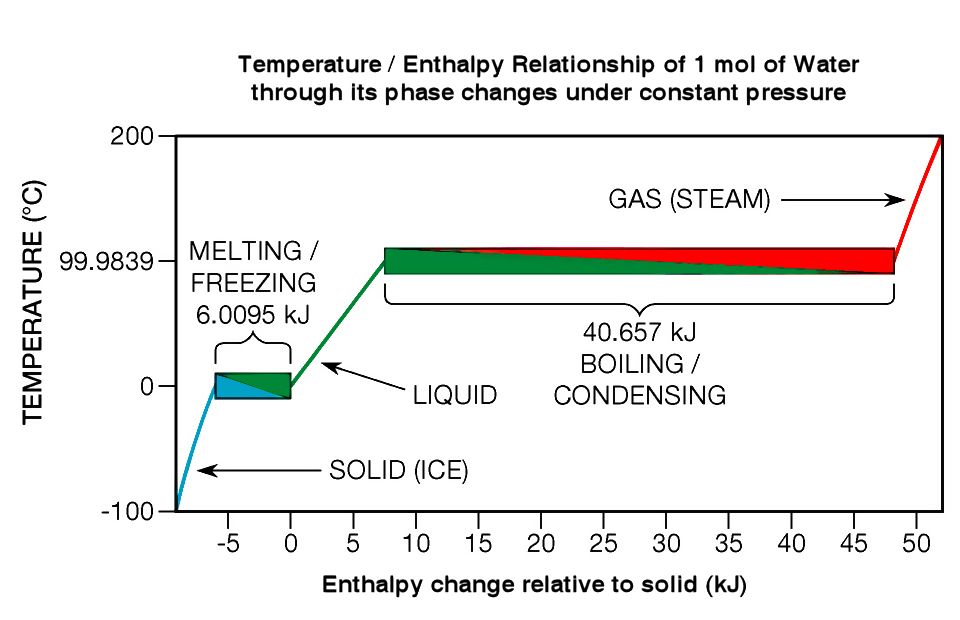# What is the Difference Between Enthalpy of Formation and Enthalpy of Reaction

The main difference between enthalpy of formation and enthalpy of reaction is that the enthalpy of formation is the enthalpy difference between the product and the elements of formation in their standard state (solid, liquid, gas), whereas enthalpy of reaction is the difference between the enthalpy of product and the enthalpy of reactants.

Almost all chemicals perform chemical reactions. When reactants react with each other to form the products in chemical reactions, we can observe both physical and chemical changes. Changes that happen during chemical reactions are changes in temperature, size, shape, state, enthalpy, etc. Enthalpy is the sum of internal energy and the product of the pressure and volume of a thermodynamic system.

### Key Areas Covered

1. What is Enthalpy
– Definition, Features
2. What is Enthalpy of Formation
– Definition, Features, Calculation
3. What is Enthalpy of Reaction
– Definition, Features, Calculation
4. Difference Between Enthalpy of Formation and Enthalpy of Reaction
– Comparison of Key Differences

### Key Terms

Enthalpy, Enthalpy of Formation, Enthalpy of Reaction## What is Enthalpy

Enthalpy is a thermodynamic property that measures the total energy in a thermodynamic system, including the internal energy and the energy associated with its pressure and volume. It is also a state function. Furthermore, enthalpy has dimensions of energy, and the units of enthalpy are Joules. Temperature, composition, and pressure determine the value of enthalpy for a particular thermodynamic system. The equation for enthalpy is H=E+PV. Here, H is the enthalpy, E is the energy, P is the pressure, and V is the volume. There are different types of enthalpy values associated with different types of reactions. Examples are enthalpy of formation, enthalpy of reaction, enthalpy of hydration, enthalpy of ionization, and enthalpy of vaporization, etc.## What is Enthalpy of Formation

Enthalpy of formation of a compound is the enthalpy change (heat given off or taken in) that occurs between the compound and its elements in their standard states in a formation reaction. To determine the enthalpy of formation, we have to first calculate the sum of the enthalpies of products and that of reactants. Then we have to find the difference between the sum of the enthalpies of products and the sum of the enthalpies of reactants.

The standard enthalpy of formation is the heat given out or taken in when one mole of the product is formed from its pure constituent elements in their normal physical state (gas, liquid, solid states). This is under standard temperature and pressure. Moreover, the standard enthalpy of formation of any element is considered zero arbitrarily. In addition, the symbol for the enthalpy of formation is ΔHf.

## What is Enthalpy of Reaction?

The enthalpy of reaction is the enthalpy change between the enthalpy of products and the enthalpy of reactants at constant pressure. To determine the enthalpy of reaction, first, calculate the sum of the enthalpies of products and the sum of the enthalpies of reactants. Then calculate the difference between the two summations to obtain the enthalpy of the reaction. The standard enthalpy of reaction is the enthalpy change occurring in a system when a chemical reaction transforms the matter, and all reactants and products are in their standard states. Moreover, the symbol for the enthalpy of reaction is ΔHr. In addition, the equation to calculate the enthalpy of the reaction is as follows:Where

Vis the stoichiometric coefficient of the products from the balanced reaction

Vr is the stoichiometric coefficient of the reactants from the balanced equation

Hf is the enthalpy of formation of products or reactants.

## Difference Between Enthalpy of Formation and Enthalpy of Reaction

### Definition

The enthalpy of formation is the enthalpy difference between the product and the elements of formation in their standard state, while the enthalpy of reaction is the enthalpy difference between the enthalpy of products and the enthalpy of reactants.

### Standard State Definition

The standard enthalpy of formation is the heat taken in or given off when one mole of the compound is formed from its pure constituent elements in its normal states under standard conditions. The enthalpy of reaction, in contrast, is the difference between the enthalpies of products and the enthalpies of reactants under standard conditions.

### Method of Calculation

To determine the enthalpy of formation, we have to first calculate the sum of the enthalpies of products and that of reactants. Then we have to find the difference between the sum of the enthalpies of products and the sum of the enthalpies of reactants. To determine the enthalpy of reaction, first, calculate the sum of the enthalpies of products and the sum of the enthalpies of reactants. Then calculate the difference between the two summations to obtain the enthalpy of the reaction.

### Conclusion

All chemicals react to form compounds, and there are changes associated with the chemical reactions thus formed. The enthalpy change is one example of such a change and simply indicates the heat taken in or given off during a chemical reaction. Enthalpy of formation is the enthalpy difference between the products and the elements of formation in their pure and standard state. But enthalpy of reaction is the difference between the enthalpy of products and the enthalpy of reactants. Thus, this is the main difference between enthalpy of formation and enthalpy of reaction.

##### Reference:

1. “Heat of Reaction.” Libre Texts – Chemistry.

##### Image Courtesy:

1. “Energy through phase changes” By Greg L at the English-language Wikipedia (CC BY-SA 3.0) via Commons Wikimedia### About the Author: Hasini A

Hasini is a graduate of Applied Science with a strong background in forestry, environmental science, chemistry, and management science. She is an amateur photographer with a keen interest in exploring the wonders of nature and science.